# Cantor manifold

(diff) ← Older revision | Latest revision (diff) | Newer revision → (diff)

An-dimensional compact space,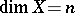, in which any partitionbetween non-empty sets has dimension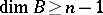. An equivalent definition is: An-dimensional Cantor manifold is an-dimensional compact spacesuch that for each representation ofas the union of two non-empty closed proper subsets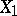and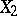,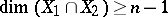. One-dimensional metrizable Cantor manifolds are one-dimensional continua or Cantor curves (cf. Cantor curve).

The concept of a Cantor manifold was introduced by P.S. Urysohn (see ). An-dimensional closed ball, and therefore an-dimensional closed manifold, are Cantor manifolds;-dimensional Euclidean space cannot be partitioned by a set of dimension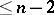(for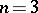, this is Urysohn's theorem, for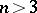, Aleksandrov's theorem). An-dimensional Cantor manifold is the common boundary of two regions of-dimensional Euclidean space, one of which is bounded (Aleksandrov's theorem). The main fact in the theory of Cantor manifolds is that every-dimensional compact space contains an-dimensional Cantor manifold (Aleksandrov's theorem).

A maximal-dimensional Cantor manifold in an-dimensional compact spaceis called a dimensional component of. An-dimensional Cantor submanifold of a compact Hausdorff spaceis contained in a unique dimensional component of. The intersection of two distinct dimensional components of an-dimensional compact Hausdorff spacehas dimension. In particular, dimensional components of a one-dimensional compact Hausdorff space are components of it. The set of dimensional components of a finite-dimensional compact metric space is finite, countable or has the cardinality of the continuum. Ifis an arbitrary dimensional component of a perfectly-normal compact spaceandis the union of all remaining dimensional components, then(Aleksandrov's theorem). In a hereditarily-normal first-countable compact Hausdorff space, a dimensional component may be contained in the union of the remaining dimensional components.

The unionof all dimensional components of an-dimensional compact spaceis called the interior dimensional kernel of the space. In view of the monotonicity of dimension, it is always true that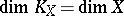and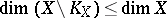whenis a perfectly-normal compact space. The setcontains no-dimensional compact set. But even for Hausdorff compacta it is not known (1978) whether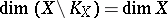. With regard to hereditarily-normal compact spaces, the interior dimensional kernel and its complement can have all permissible dimensions; that is to say, assuming the validity of the continuum hypothesis, for any triple of integers,and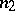with,and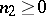, there exists a hereditarily-normal compact spaceof dimensionsuch that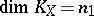and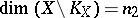.

If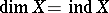, then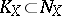(as defined by Urysohn) is the inductive dimensional kernel, that is, the set of allfor which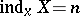. The inductive dimensional kernel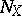of a compact metric setis always an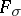set. It is not known whether the same holds for the interior dimensional kernel. For compact Hausdorff spaces however, neither the inductive dimensional kernel nor the interior dimensional kernel need be anset. At each point,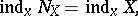ifis compact metric (Menger's theorem). Therefore for an arbitrary compact metric space,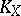is everywhere dense in. This does not carry over to arbitrary compact Hausdorff spaces. It remains an open question (1978) whether a point is contained in the inductive dimensional kernel along with some non-degenerate continuum.

A finite-dimensional continuumwhose interior dimensional kernelis everywhere dense inis called a generalized Cantor manifold. The common boundary of two open subsets of-dimensional Euclidean space is an-dimensional generalized Cantor manifold. In a metrizable-dimensional generalized Cantor manifoldthere may be an everywhere-dense set of pointsfor which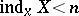. Neither products nor continuous mappings preserve the property of being a generalized Cantor manifold. The same is true concerning the property of being a Cantor manifold.

A compact spaceis called an infinite-dimensional Cantor manifold if there is no method of partitioning it by a weakly infinite-dimensional closed subset.

How to Cite This Entry:
Cantor manifold. Encyclopedia of Mathematics. URL: http://encyclopediaofmath.org/index.php?title=Cantor_manifold&oldid=14407
This article was adapted from an original article by V.V. Fedorchuk (originator), which appeared in Encyclopedia of Mathematics - ISBN 1402006098. See original article